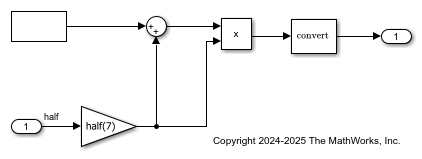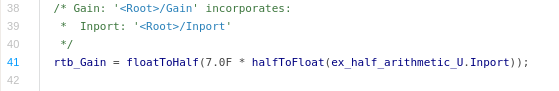## The Half-Precision Data Type in Simulink

Signals and block outputs can specify a half-precision data type. The half-precision data type is supported for simulation and code generation for parameters and a subset of blocks.

### Math Operations in Half-Precision

In Simulink®, half-precision inputs to blocks performing arithmetic operations, relational operations, and binary operations are always cast to single precision, and the operation is performed in single precision. If the output data type of the block is set to `half`, the output of the block is cast back to a half-precision data type.

In MATLAB®, however, some functions perform arithmetic operations with half-precision inputs by emulating the half-precision floating-point math. For example, in MATLAB, the following code is performed using half-precision floating-point arithmetic.

`y = mod(half(u1), half(u2))`

In Simulink, using the `mod` function of the Math Function block, the same operation would be performed by casting the inputs to single precision and carrying out the operation in single-precision floating-point math. The result of the arithmetic operations is then cast back to half precision.

`y = half(mod(single(half(u1)), single(half(u2))))`

### Software Features Supported for Half Precision

• The half-precision data type is supported for simulation in Normal, Accelerator, and Rapid Accelerator modes. The half-precision data type is also supported for SIL, PIL, and external modes.

• Half precision is supported for C/C++ code generation for `.ert` targets.

In the generated code, half-precision variables are stored in a class emulating the bit pattern of the value.

• For embedded hardware targets that natively support special types for half precision, native half-precision C code generation is supported. For more information, see Generate Native Half-Precision C Code from Simulink Models.

• HDL code generation using HDL Coder™.

For more information, see Getting Started with HDL Coder Native Floating-Point Support (HDL Coder).

• MATLAB System block supports half-precision data type with real values.

• In Simulink, the half-precision data type only supports real values. Complex values cannot have a half-precision data type.

#### Blocks Supported for Half Precision

To view the blocks that support half precision, at the command line, type:

`showblockdatatypetable`

Blocks that support half precision display an `X` in the column labeled Half.

### Generate Code for Half Precision Systems

You can generate C code targeting `.ert` targets for Simulink models using the half-precision data type. Code generation for `.ert` targets requires an Embedded Coder® license.

Open the `ex_half_arithmetic` model. The model performs several arithmetic operations. All parameter values and output data types specify a half-precision data type.

```open_system('ex_half_arithmetic'); ```To generate C code for the model, press Ctrl+B. In the code generation report, open the `ex_half_arithmetic.c` file. Half-precision variables are types in the generated code as `real16_T`. For example, see the `rtb_Gain` variable.In the generated code, half-precision variables are stored in a struct emulating the bit pattern of the value.

Half-precision input variables to arithmetic operations are cast to single precision, and the arithmetic operation is performed in single precision. If the output data type of the block is set to `half`, the result of the operation is cast back to a half-precision data type. For example, see the code computing the output of the Gain block.